Next: Method of determination of Up: Introduction - existing methods Previous: Introduction - existing methods

## Equation and Scherrer constant for polidisperse powders

Scherrer equation: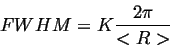constraints diffraction peak width FWHM and average size of crystallites <R> in the powder by the constant K, known as a Scherrer constant. It has been found that peak width, thus Scherrer constant, depends on dispersion of crystallite sizes of the powder :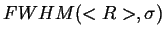=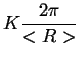=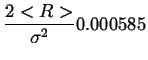(1)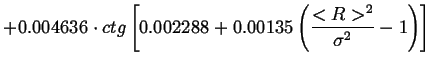Figure 1 shows Scherrer constant's dependence on normalized Grain Size Distribution width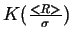.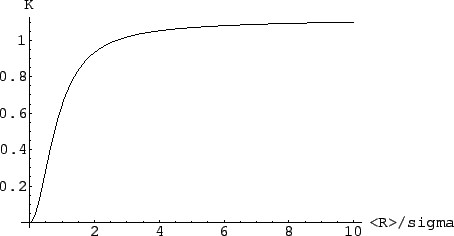In practice, one may estimate lowest value of K as c.a. 0.5 since it corresponds to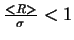, which means dispersion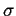larger than average grain size <R>. Highest value of K corresponds to the powder containing grains of identical sizes (mono-dispersive).

Sense of the Fig. 1 may be summarized as follows: value of the Scherrer constant depends on normalized Grain Size Distribution width. Moreover, in real cases this dependence is very strong. E.g. nanocrystalline powders of SiC after synthesis (with no segregation applied) have ratio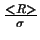in range of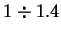, which belongs to the region of the steepest slope at Fig.1. Ratiomaintains its value for the materials of the same origin, disregarding actual size of the crystallites synthesized. This ratio changes after intentional segregation only.

Let's imagine we have synthesized a crystalline powder having some distribution of sizes. Using a system of sieves we segregate entire powder into fractions with more and more precisely defined crystal sizes. Simultaneously, using powder diffraction and Scherrer equation we want to determine average grain size in subsequent fractions. Unfortunately, it turns out that we need to use a different Scherrer constant for each fraction: lower at the beginning of the segregation (while the powder is still a mixture of small and big crystals, say K=0.5), higher at the end (for mono-dispersive fractions, say K=1.1), since K depends on width of the Grain Size Distribution.

Attempts of precise determination of the grain size using this method are questionable as for calculation of exact <R> value one needs to know exact value of K, which depends on width of Grain Size Distribution that is the quantity we're actually looking for. Since K varies in wide range from c.a. 0.5 to c.a. 1.1, so as much as two times, this is the possible deviation of the average grain size obtained.

It must be stressed that aside from errors of order - typically - tens of %, Scherrer method is certainly the best existing, the simplest and most elegant. However, the difficulties described show that it would be nice to have a method of determination both parameters of the Grain Size Distribution: it's average and dispersion. Not only to reduce deviation of <R> but mostly for determination of dispersion of crystallite sizes.Next: Method of determination of Up: Introduction - existing methods Previous: Introduction - existing methods
roman pielaszek 2003-03-17